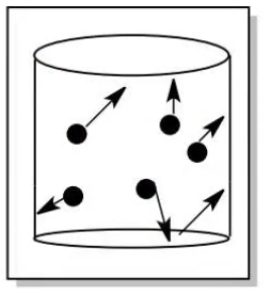# Problem: The temperature of a gas is a measure of a. the kinetic energy of the gas particles. b. the volumes of the gas particles.c. the amount of space between gas particles. d. the number of gas particles.

###### FREE Expert Solution

We´re asked to determine which of the given options defines the temperature of a gas.

Temperature is a measure of thermal energy in a substance, and it’s independent of the amount of matter.

Imagine a container filled with black balls.The black balls represent individual gas particles.

In each black ball, there are arrows pointing in different directions.

86% (85 ratings)###### Problem Details

The temperature of a gas is a measure of

a. the kinetic energy of the gas particles.

b. the volumes of the gas particles.

c. the amount of space between gas particles.

d. the number of gas particles.

Frequently Asked Questions

What scientific concept do you need to know in order to solve this problem?

Our tutors have indicated that to solve this problem you will need to apply the Kinetic Molecular Theory concept. You can view video lessons to learn Kinetic Molecular Theory. Or if you need more Kinetic Molecular Theory practice, you can also practice Kinetic Molecular Theory practice problems.